• ## python生成稀疏矩阵

千次阅读 2018-09-22 17:03:25
from scipy.sparse import coo_matrix row = [2,2,3,2] col = [3,4,2,3] c = coo_matrix((data,(row,col)),shape=(5,6))

from scipy.sparse import coo_matrix

row = [2,2,3,2]

col = [3,4,2,3]

c = coo_matrix((data,(row,col)),shape=(5,6))

展开全文• 喜欢(doc1 , term1 ,12)(doc1, term 22, 2)..(docn,term1 , 10)该矩阵可以被视为稀疏矩阵，因为每个文档都包含很少的具有非零值的项。我如何使用numpy从稀疏矩阵创建密集矩阵，因为我必须使用余弦相似度...
我有一个Sqlite数据库，其中包含以下类型的架构:termcount(doc_num, term , count)该表包含术语及其在文档中的各自计数。喜欢(doc1 , term1 ,12)(doc1, term 22, 2)..(docn,term1 , 10)该矩阵可以被视为稀疏矩阵，因为每个文档都包含很少的具有非零值的项。我如何使用numpy从稀疏矩阵创建密集矩阵，因为我必须使用余弦相似度来计算文档之间的相似度。这个密集的矩阵看起来像一个表格，第一列为docid，所有术语将列为第一行，其余单元格将包含计数。参考方案from scipy.sparse import csr_matrixA = csr_matrix([[1,0,2],[0,3,0]])>>>A<2x3 sparse matrix of type ''with 3 stored elements in Compressed Sparse Row format>>>> A.todense()matrix([[1, 0, 2],[0, 3, 0]])>>> A.toarray()array([[1, 0, 2],[0, 3, 0]])这是一个如何将稀疏矩阵转换为取自scipy的密集矩阵的示例Python GPU资源利用 - python我有一个Python脚本在某些深度学习模型上运行推理。有什么办法可以找出GPU资源的利用率水平？例如，使用着色器，float16乘法器等。我似乎在网上找不到太多有关这些GPU资源的文档。谢谢！ 参考方案 您可以尝试在像Renderdoc这样的GPU分析器中运行pyxthon应用程序。它将分析您的跑步情况。您将能够获得有关已使用资源，已用缓冲区，不同渲染状态上…Python:图像处理可产生皱纹纸效果 - python也许很难描述我的问题。我正在寻找Python中的算法，以在带有某些文本的白色图像上创建皱纹纸效果。我的第一个尝试是在带有文字的图像上添加一些真实的皱纹纸图像（具有透明度）。看起来不错，但副作用是文本没有真正起皱。所以我正在寻找更好的解决方案，有什么想法吗？谢谢 参考方案 除了使用透明性之外，假设您有两张相同尺寸的图像，一张在皱纹纸上明亮，一张在白色背景上有深…Python uuid4，如何限制唯一字符的长度 - python在Python中，我正在使用uuid4（）方法创建唯一的字符集。但是我找不到将其限制为10或8个字符的方法。有什么办法吗？uuid4()ffc69c1b-9d87-4c19-8dac-c09ca857e3fc谢谢。 参考方案 尝试：x = uuid4() str(x)[:8] 输出："ffc69c1b" Is there a way to…Python sqlite3数据库已锁定 - python我在Windows上使用Python 3和sqlite3。我正在开发一个使用数据库存储联系人的小型应用程序。我注意到，如果应用程序被强制关闭（通过错误或通过任务管理器结束），则会收到sqlite3错误（sqlite3.OperationalError：数据库已锁定）。我想这是因为在应用程序关闭之前，我没有正确关闭数据库连接。我已经试过了： connectio…Python:如何停止多线程的numpy？ - python我知道这似乎是一个荒谬的问题，但是我必须在与部门中其他人共享的计算服务器上定期运行作业，当我开始10个作业时，我真的希望它只占用10个核心而不是更多;我不在乎每次运行一个内核所需的时间是否更长:我只是不想让它侵犯其他人的领土，这将需要我放弃工作等等。我只想拥有10个核心，仅此而已。更具体地说，我在基于Python 2.7.3和numpy 1.6.1的Redh…
展开全文• 稀疏矩阵生成，可视化
生成随机矩阵
import numpy as np
import scipy.sparse as ss

# 生成随机稀疏矩阵
num_col = 20
num_row = 10
num_ele = 40
a = [np.random.randint(0,num_row) for _ in range(num_ele)]
b = [np.random.randint(0,num_col) for _ in range(num_ele-num_col)] + [i for i in range(num_col)]  # 保证每一列都有值，不会出现全零列
c = [np.random.rand() for _ in range(num_ele)]
rows, cols, v = np.array(a), np.array(b), np.array(c)
sparseX = ss.coo_matrix((v,(rows,cols)))
X = sparseX.todense()

fig = plt.figure()
ax.matshow(X, vmin=0, vmax=1, cmap='magma')
ax.set_xticks([])
ax.set_yticks([])
plt.show()常用 colormap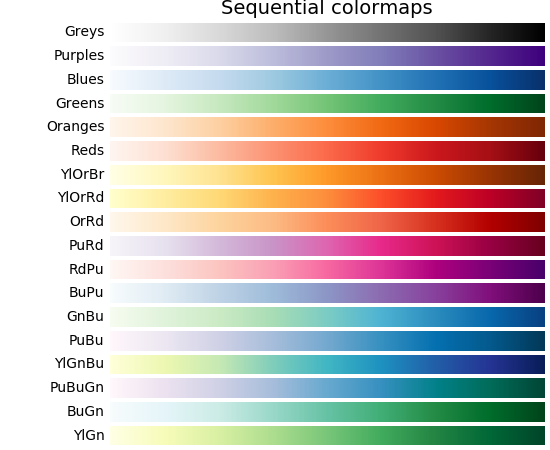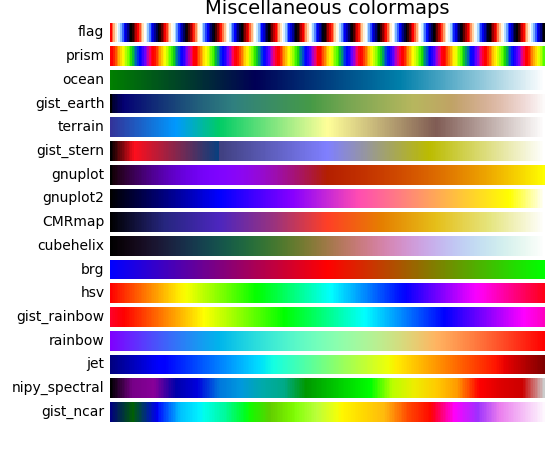生成稀疏编码信号
from sklearn.datasets import make_sparse_coded_signal

n_samples, n_components, n_features = 5, 10, 8
n_nonzero_coefs = 3

# Y = DX
# |x_i|_0 = n_nonzero_coefs

Y, D, X = make_sparse_coded_signal(n_samples=n_samples,
n_components=n_components,
n_features=n_features,
n_nonzero_coefs=n_nonzero_coefs,
random_state=42)

这是字典学习中处理的问题：
$Y = DX$
$X$ 为原始信号 $Y$ 的稀疏编码。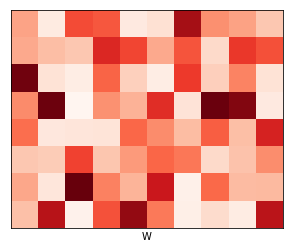展开全文• ## python scipy 稀疏矩阵详解

万次阅读 多人点赞 2019-08-25 16:40:38
文章目录稀疏矩阵格式coo_matrixcsr_matrixcsc_matrixlil_matrixdok_matrixdia_matrixbsr_matrix实用函数经验总结 稀疏矩阵格式 coo_matrix   coo_matrix是最简单的稀疏矩阵存储方式，采用三元组(row, col, data)...
文章目录稀疏矩阵格式coo_matrixcsr_matrixcsc_matrixlil_matrixdok_matrixdia_matrixbsr_matrix实用函数经验总结参考
稀疏矩阵格式
coo_matrix
coo_matrix是最简单的稀疏矩阵存储方式，采用三元组(row, col, data)(或称为ijv format)的形式来存储矩阵中非零元素的信息。在实际使用中，一般coo_matrix用来创建矩阵，因为coo_matrix无法对矩阵的元素进行增删改操作；创建成功之后可以转化成其他格式的稀疏矩阵（如csr_matrix、csc_matrix）进行转置、矩阵乘法等操作。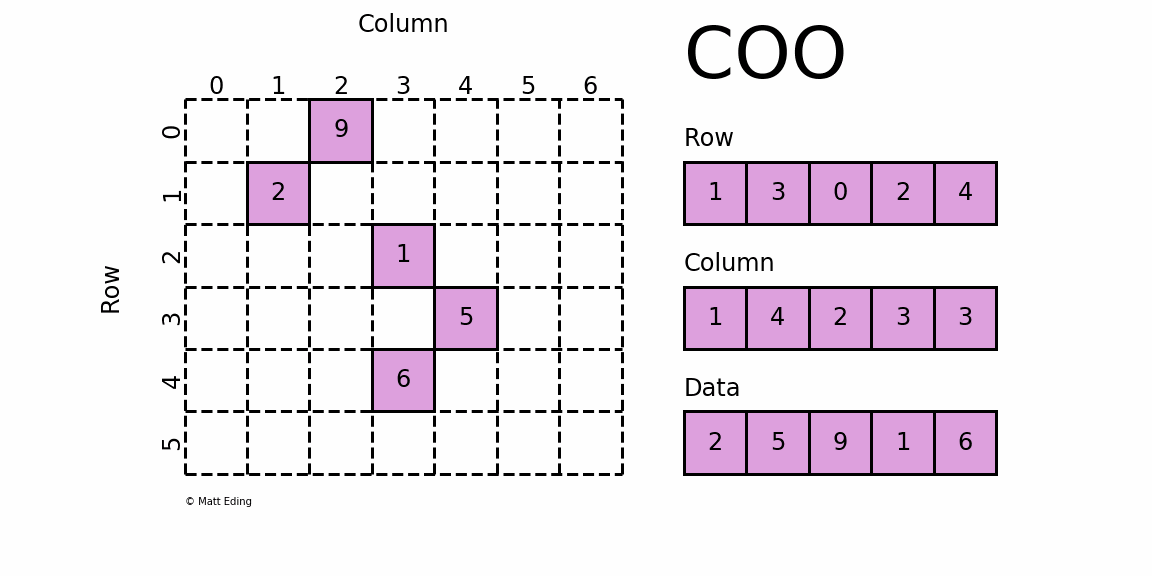​		coo_matrix可以通过四种方式实例化，除了可以通过coo_matrix(D), D代表密集矩阵；coo_matrix(S), S代表其他类型稀疏矩阵或者coo_matrix((M, N), [dtype])构建一个shape为M*N的空矩阵，默认数据类型是d，还可以通过(row, col, data)三元组初始化：
>>> import numpy as np
>>> from scipy.sparse import coo_matrix

>>> _row  = np.array([0, 3, 1, 0])
>>> _col  = np.array([0, 3, 1, 2])
>>> _data = np.array([4, 5, 7, 9])
>>> coo = coo_matrix((_data, (_row, _col)), shape=(4, 4), dtype=np.int)
>>> coo.todense()  # 通过toarray方法转化成密集矩阵(numpy.matrix)
>>> coo.toarray()  # 通过toarray方法转化成密集矩阵(numpy.ndarray)
array([[4, 0, 9, 0],
[0, 7, 0, 0],
[0, 0, 0, 0],
[0, 0, 0, 5]])

​		上面通过triplet format的形式构建了一个coo_matrix对象，我们可以看到坐标点(0,0)对应值为4，坐标点(1,1)对应值为7等等，这就是coo_matrix。coo_matrix对象有很多方法，大多数是elementwise的操作函数；coo_matrix对象有以下属性：

dtype dtype
矩阵中元素的数据类型

shape 2-tuple
获取矩阵的shape

ndim int
获取矩阵的维度，当然值是2咯

nnz
存储值的个数，包括显示声明的零元素(注意)

data
稀疏矩阵存储的值，是一个一维数组，即上面例子中的_data

row
与data同等长度的一维数组，表征data中每个元素的行号

col
与data同等长度的一维数组，表征data中每个元素的列号
​	在实际应用中，coo_matrix矩阵文件通常存成以下形式，表示稀疏矩阵是coo_matrix(coordinate)，由13885行1列组成，共有949个元素值为非零，数据类型为整形。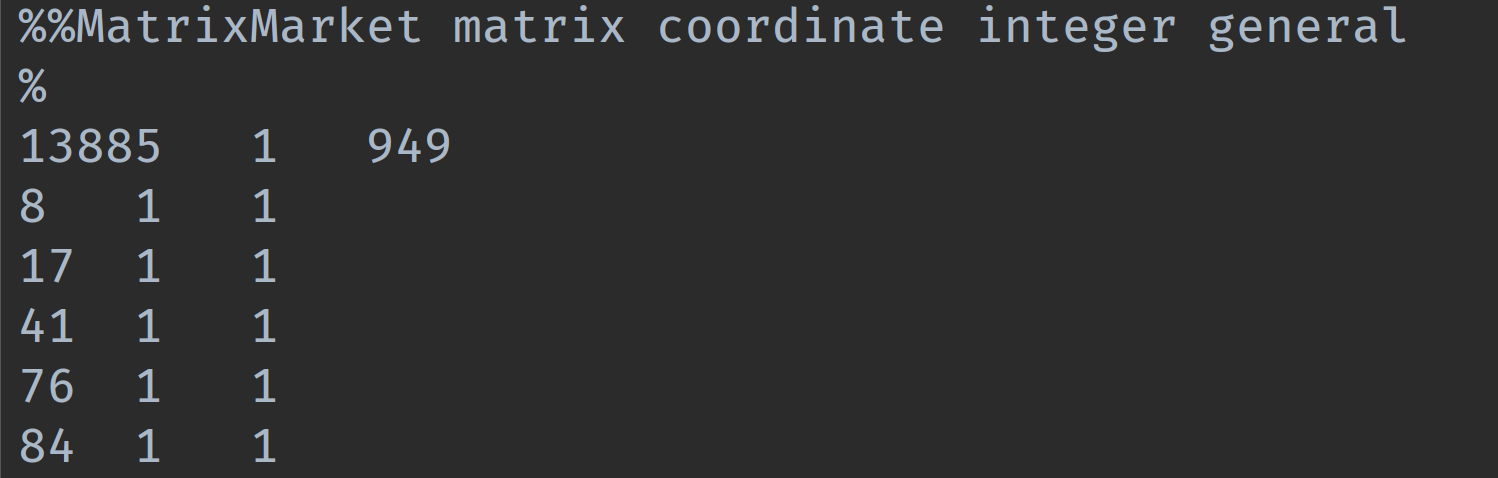​		下面给出coo_matrix矩阵文件读写示例代码，mmread()用于读取稀疏矩阵，mmwrite()用于写入稀疏矩阵，mminfo()用于查看稀疏矩阵文件元信息。(这三个函数的操作不仅仅限于coo_matrix)
from scipy.io import mmread, mmwrite, mminfo

HERE = dirname(__file__)
coo_mtx_path = join(HERE, 'data/matrix.mtx')
print(mminfo(coo_mtx_path))
# (13885, 1, 949, 'coordinate', 'integer', 'general')
# (rows, cols, entries, format, field, symmetry)
mmwrite(join(HERE, 'data/saved_mtx.mtx'), coo_mtx)

coo_matrix的优点：

有利于稀疏格式之间的快速转换（tobsr()、tocsr()、to_csc()、to_dia()、to_dok()、to_lil()）
允许又重复项（格式转换的时候自动相加）
能与CSR / CSC格式的快速转换

coo_matrix的缺点：

不能直接进行算术运算

csr_matrix
​		csr_matrix，全称Compressed Sparse Row matrix，即按行压缩的稀疏矩阵存储方式，由三个一维数组indptr, indices, data组成。这种格式要求矩阵元按行顺序存储，每一行中的元素可以乱序存储。那么对于每一行就只需要用一个指针表示该行元素的起始位置即可。indptr存储每一行数据元素的起始位置，indices这是存储每行中数据的列号，与data中的元素一一对应。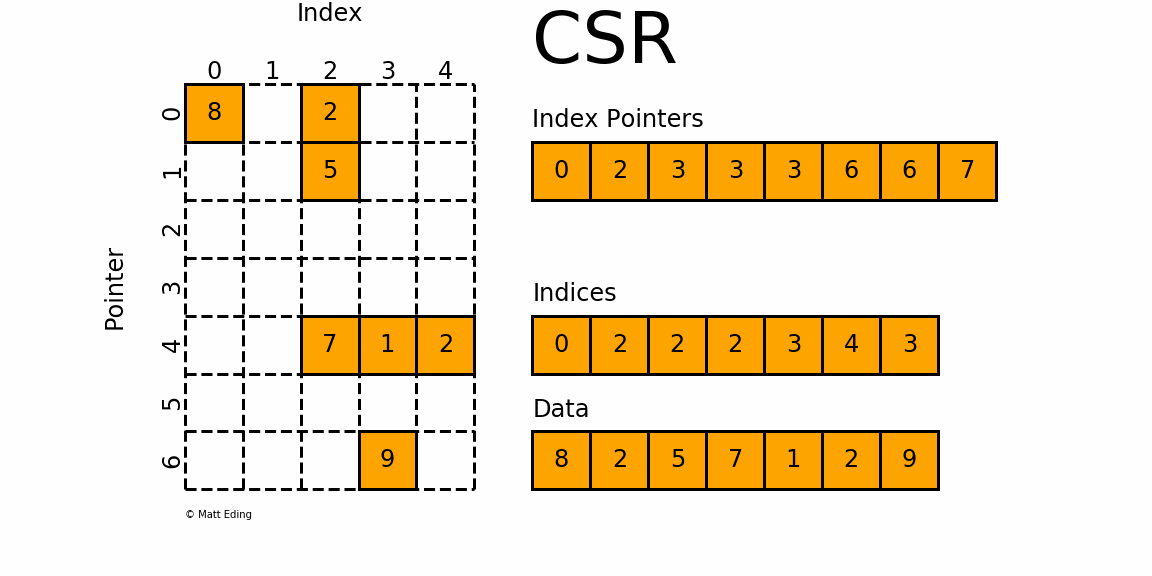​		csr_matrix可用于各种算术运算：它支持加法，减法，乘法，除法和矩阵幂等操作。其有五种实例化方法，其中前四种初始化方法类似coo_matrix，即通过密集矩阵构建、通过其他类型稀疏矩阵转化、构建一定shape的空矩阵、通过(row, col, data)构建矩阵。其第五种初始化方式这是直接体现csr_matrix的存储特征：csr_matrix((data, indices, indptr), [shape=(M, N)])，意思是，矩阵中第i行非零元素的列号为indices[indptr[i]:indptr[i+1]]，相应的值为data[indptr[i]:indptr[i+1]]
举个例子：
>>> import numpy as np
>>> from scipy.sparse import csr_matrix

>>> indptr = np.array([0, 2, 3, 6])
>>> indices = np.array([0, 2, 2, 0, 1, 2])
>>> data = np.array([1, 2, 3, 4, 5, 6])
>>> csr = csr_matrix((data, indices, indptr), shape=(3, 3)).toarray()
array([[1, 0, 2],
[0, 0, 3],
[4, 5, 6]])

​		csr_matrix同样有很多方法，其中tobytes()，tolist(), tofile()，tostring()值得注意，其他具体参考官方文档，csr_matrix对象属性前五个同coo_matrix，另外还有属性如下：

indices
与属性data一一对应，元素值代表在某一行的列号

indptr
csr_matrix各行的起始值，length(csr_object.indptr) == csr_object.shape + 1

has_sorted_indices
判断每一行的indices是否是有序的，返回bool值

csr_matrix的优点：

高效的算术运算CSR + CSR，CSR * CSR等
高效的行切片
快速矩阵运算

csr_matrix的缺点：

列切片操作比较慢（考虑csc_matrix）
稀疏结构的转换比较慢（考虑lil_matrix或doc_matrix）

csc_matrix
​		csc_matrix和csr_matrix正好相反，即按列压缩的稀疏矩阵存储方式，同样由三个一维数组indptr, indices, data组成，如下图所示：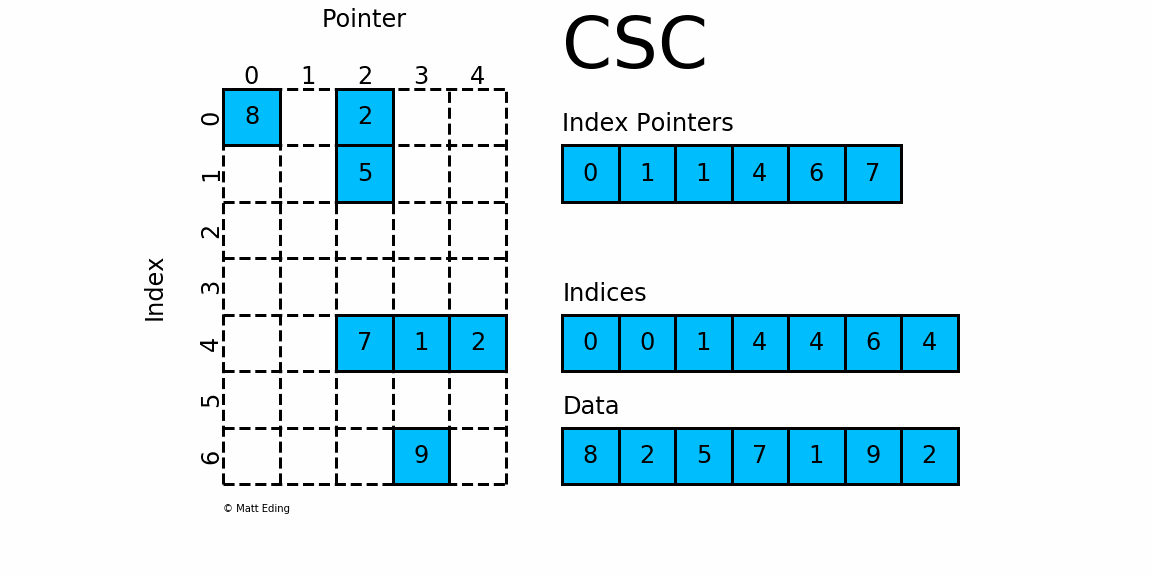​		其实例化方式、属性、方法、优缺点和csr_matrix基本一致，这里不再赘述，它们之间唯一的区别就是按行或按列压缩进行存储。而这一区别决定了csr_matrix擅长行操作；csc_matrix擅长列操作，进行运算时需要进行合理存储结构的选择。
lil_matrix
​		lil_matrix，即List of Lists format，又称为Row-based linked list sparse matrix。它使用两个嵌套列表存储稀疏矩阵：data保存每行中的非零元素的值，rows保存每行非零元素所在的列号(列号是顺序排序的)。这种格式很适合逐个添加元素，并且能快速获取行相关的数据。其初始化方式同coo_matrix初始化的前三种方式：通过密集矩阵构建、通过其他矩阵转化以及构建一个一定shape的空矩阵。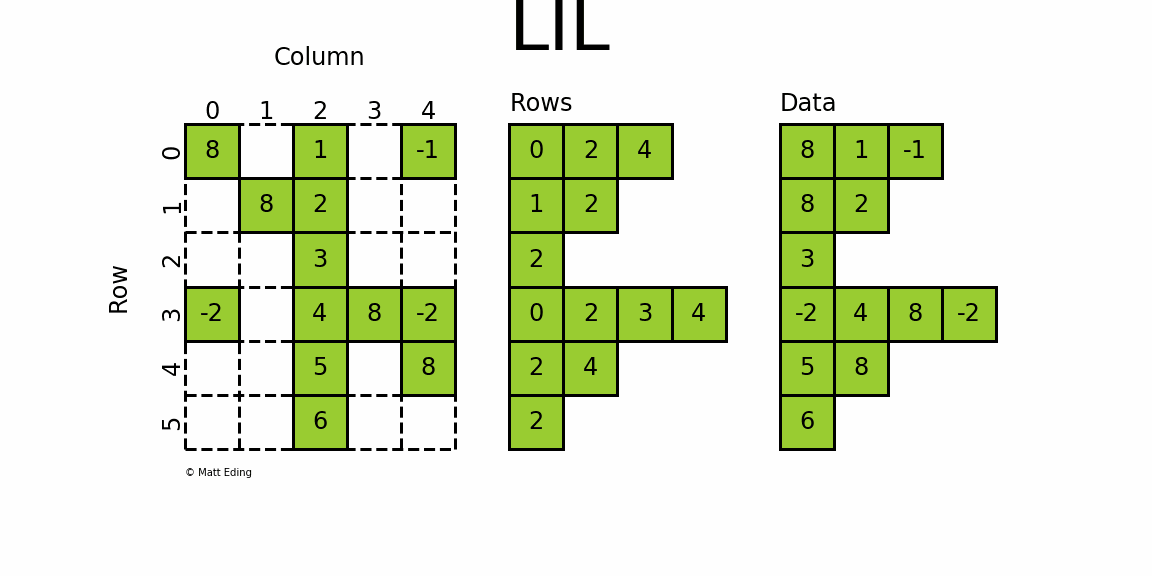​		lil_matrix可用于算术运算：支持加法，减法，乘法，除法和矩阵幂。其属性前五个同coo_matrix，另外还有rows属性，是一个嵌套List，表示矩阵每行中非零元素的列号。LIL matrix本身的设计是用来方便快捷构建稀疏矩阵实例，而算术运算、矩阵运算则转化成CSC、CSR格式再进行，构建大型的稀疏矩阵还是推荐使用COO格式。
LIL format优点

支持灵活的切片操作行切片操作效率高，列切片效率低
稀疏矩阵格式之间的转化很高效（tobsr()、tocsr()、to_csc()、to_dia()、to_dok()、to_lil()）

LIL format缺点

加法操作效率低 (consider CSR or CSC)
列切片效率低(consider CSC)
矩阵乘法效率低 (consider CSR or CSC)

dok_matrix
​		dok_matrix，即Dictionary Of Keys based sparse matrix，是一种类似于coo matrix但又基于字典的稀疏矩阵存储方式，key由非零元素的的坐标值tuple(row, column)组成，value则代表数据值。dok matrix非常适合于增量构建稀疏矩阵，并一旦构建，就可以快速地转换为coo_matrix。其属性和coo_matrix前四项同；其初始化方式同coo_matrix初始化的前三种：通过密集矩阵构建、通过其他矩阵转化以及构建一个一定shape的空矩阵。对于dok matrix，可用于算术运算：它支持加法，减法，乘法，除法和矩阵幂；允许对单个元素进行快速访问（ O(1) ）; 不允许重复。
>>> import numpy as np
>>> from scipy.sparse import dok_matrix

>>> np.random.seed(10)
>>> matrix = random(3, 3, format='dok', density=0.4)
>>> matrix[1, 1] = 33
>>> matrix[2, 1] = 10
>>> matrix.toarray()
array([[ 0.        ,  0.        ,  0.        ],
[ 0.        , 33.        ,  0.        ],
[ 0.19806286, 10.        ,  0.22479665]])
>>> dict(matrix)
{(2, 0): 0.19806286475962398, (2, 1): 10.0, (2, 2): 0.22479664553084766, (1, 1): 33.0}

>>> isinstance(matrix, dict)
True

​		在上面代码最后可以看到，实际上dok_matrix实例也是dict实例，在实现上继承了dict类。
dia_matrix
​		dia_matrix，全称Sparse matrix with DIAgonal storage，是一种对角线的存储方式。如下图中，将稀疏矩阵使用offsets和data两个矩阵来表示。offsets表示data中每一行数据在原始稀疏矩阵中的对角线位置k（k>0, 对角线往右上角移动；k<0, 对角线往左下方移动；k=0，主对角线）。该格式的稀疏矩阵可用于算术运算：它们支持加法，减法，乘法，除法和矩阵幂。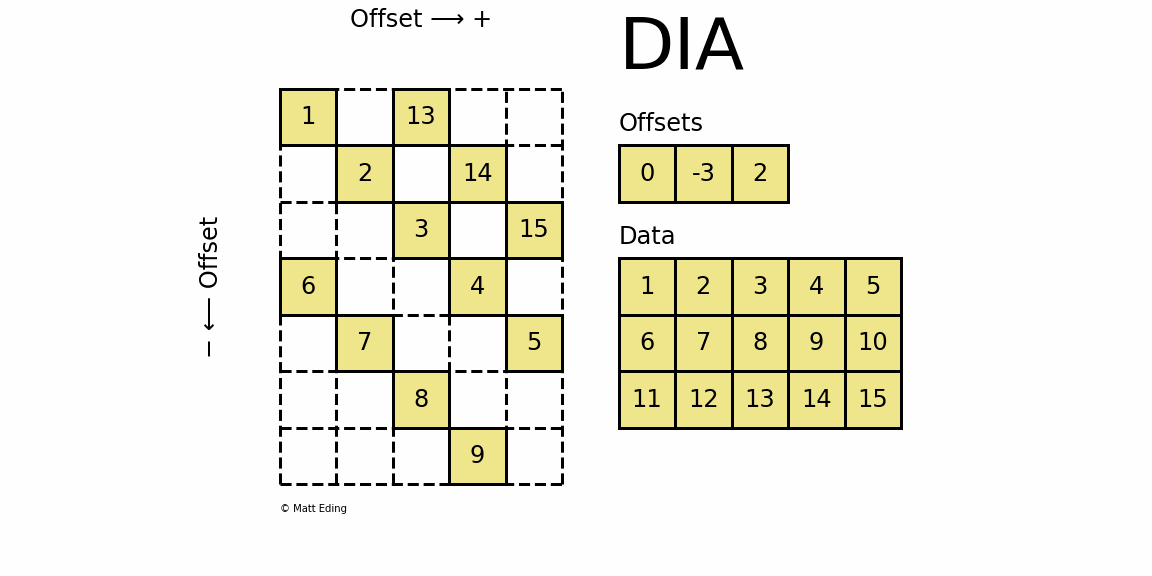​		dia_matrix五个属性同coo matrix, 另外还有属性offsets；dia_matrix有四种初始化方式，其中前三种初始化方式同coo_matrix前三种初始化方式，即：通过密集矩阵构建、通过其他矩阵转化以及构建一个一定shape的空矩阵。第四种初始化方式如下：
dia_matrix((data, offsets), shape=(M, N)) ,
​												其中，data[k,:]存储着稀疏矩阵offsets[k]对角线上的值
>>> data = np.arange(15).reshape(3, -1) + 1
>>> offsets = np.array([0, -3, 2])
>>> dia = sparse.dia_matrix((data, offsets), shape=(7, 5))
>>> dia.toarray()
array([[ 1,  0, 13,  0,  0],
[ 0,  2,  0, 14,  0],
[ 0,  0,  3,  0, 15],
[ 6,  0,  0,  4,  0],
[ 0,  7,  0,  0,  5],
[ 0,  0,  8,  0,  0],
[ 0,  0,  0,  9,  0]])

​																																							不是很常用,了解即可
bsr_matrix
​		bsr_matrix，全称Block Sparse Row matrix，这种压缩方式极其类似CSR格式，但使用分块的思想对稀疏矩阵进行按行压缩。所以，BSR适用于具有dense子矩阵的稀疏矩阵。该种矩阵有五种初始化方式，分别如下：

bsr_matrix(D, [blocksize=(R,C)])
D是一个M*N的二维dense矩阵；blocksize需要满足条件：M % R = 0和N % C = 0，若不给定该参数，内部将会应用启发式的算法自动决定一个合适的blocksize.

bsr_matrix(S, [blocksize=(R,C)])
S是指其他类型的稀疏矩阵

bsr_matrix((M, N), [blocksize=(R,C), dtype])
构建一个shape为M*N的空矩阵

bsr_matrix((data, ij), [blocksize=(R,C), shape=(M, N)])
data 和ij 满足条件： a[ij[0, k], ij[1, k]] = data[k]

bsr_matrix((data, indices, indptr), [shape=(M, N)])
data.shape一般是k*R*C，其中R、C分别代表block的行和列长，k代表有几个小block矩阵；第i行的块列索引存储在indices[indptr[i]:indptr[i+1]]，其值是data[ indptr[i]: indptr[i+1] ]。

bsr_matrix可用于算术运算：支持加法，减法，乘法，除法和矩阵幂。如下面的例子，对于许多稀疏算术运算，BSR比CSR和CSC更有效：
>>> from scipy.sparse import bsr_matrix
>>> import numpy

>>> indptr = np.array([0, 2, 3, 6])
>>> indices = np.array([0, 2, 2, 0, 1, 2])
>>> data = np.array([1, 2, 3, 4, 5, 6]).repeat(4).reshape(6, 2, 2)

>>> bsr_matrix((data,indices,indptr), shape=(6, 6)).toarray()
array([[1, 1, 0, 0, 2, 2],
[1, 1, 0, 0, 2, 2],
[0, 0, 0, 0, 3, 3],
[0, 0, 0, 0, 3, 3],
[4, 4, 5, 5, 6, 6],
[4, 4, 5, 5, 6, 6]])

​																													可以通过热图观察矩阵有没有明显分块模式再决定使不使用该方式
bsr matrix对象拥有9个属性，前四个属性与coo matrix相同，另外还有以下属性(注意csr matrix和bsr matrix之间的区别与联系)：

data
即稀疏矩阵的数组，data.shape一般是k*R*C

indices
与属性data中的k个二维矩阵一一对应，元素值代表在某一行的列号

indptr
bsr各行起始起始值

blocksize
即tuple(R,C)

has_sorted_indices
判断每一行的indices是否是有序的，返回bool值

实用函数

构造特殊稀疏矩阵

​		scipy.sparse模块还包含一些便捷函数，用于快速构建单位矩阵、对角矩阵等，下面做一个简单的汇总：

方法
用途

identity(n[, dtype, format])
生成稀疏单位矩阵

kron(A, B[, format])
sparse matrices A 和 B的克罗内克积

kronsum(A, B[, format])
sparse matrices A 和 B的克罗内克和

diags(diagonals[, offsets, shape, format, dtype])
构建稀疏对角阵

spdiags(data, diags, m, n[, format])
构建稀疏对角阵，同上，但不可指定shape

block_diag(mats[, format, dtype])
mats为iterable, 包含多个矩阵，根据mats构建块对角稀疏矩阵。

tril(A[, k, format])
以稀疏格式返回矩阵的下三角部分

triu(A[, k, format])
以稀疏格式返回矩阵的上三角部分

bmat(blocks[, format, dtype])
从稀疏子块构建稀疏矩阵

hstack(blocks[, format, dtype])
水平堆叠稀疏矩阵(column wise)

vstack(blocks[, format, dtype])
垂直堆叠稀疏矩阵 (row wise)

rand(m, n[, density, format, dtype, …])
使用均匀分布的值生成给定形状和密度的稀疏矩阵

random(m, n[, density, format, dtype, …])
使用随机分布的值生成给定形状和密度的稀疏矩阵

eye(m[, n, k, dtype, format])
生成稀疏单位对角阵（默认DIAgonal format）

​		scipy.sparse.bmat举例：
In : A = np.arange(8).reshape(2, 4)

In : T = np.tri(5, 4)

In : L = [ * 4] * 2

In : I = sparse.identity(4)

In : Z = sparse.coo_matrix((2, 3))

In : sp.bmat([[   A,    Z,    L],
...:          [None, None,    I],
...:          [   T, None, None]], dtype=int)
Out:
<11x11 sparse matrix of type '<class 'numpy.int64'>'
with 33 stored elements in COOrdinate format>

In : _.toarray()  # ipython previous output
Out:
array([[0, 1, 2, 3, 0, 0, 0, 8, 8, 8, 8],
[4, 5, 6, 7, 0, 0, 0, 8, 8, 8, 8],
[0, 0, 0, 0, 0, 0, 0, 1, 0, 0, 0],
[0, 0, 0, 0, 0, 0, 0, 0, 1, 0, 0],
[0, 0, 0, 0, 0, 0, 0, 0, 0, 1, 0],
[0, 0, 0, 0, 0, 0, 0, 0, 0, 0, 1],
[1, 0, 0, 0, 0, 0, 0, 0, 0, 0, 0],
[1, 1, 0, 0, 0, 0, 0, 0, 0, 0, 0],
[1, 1, 1, 0, 0, 0, 0, 0, 0, 0, 0],
[1, 1, 1, 1, 0, 0, 0, 0, 0, 0, 0],
[1, 1, 1, 1, 0, 0, 0, 0, 0, 0, 0]])


稀疏矩阵类型判断
​	scipy.sparse模块还包含一些判断稀疏矩阵类型的函数，这里需要注意的是，issparse() 和 isspmatrix() 是相同的函数，也许是由于历史原因保留下来了两个。

isspars(x)
isspmatrix(x)
isspmatrix_csc(x)
isspmatrix_csr(x)
isspmatrix_bsr(x)
isspmatrix_lil(x)
isspmatrix_dok(x)
isspmatrix_coo(x)
isspmatrix_dia(x)

稀疏矩阵存取

load_npz(file) 从.npz文件中读取稀疏矩阵
save_npz(file, matrix[,compressed]) 将稀疏矩阵写入.npz文件中

其他

find(A) 返回稀疏矩阵中非零元素的索引以及值

经验总结

要有效地构造矩阵，请使用dok_matrix或lil_matrix
lil_matrix类支持基本切片和花式索引，其语法与NumPy Array类似；lil_matrix形式是基于row的，因此能够很高效的转为csr，但是转为csc效率相对较低。

强烈建议不要直接使用NumPy函数运算稀疏矩阵
如果你想将NumPy函数应用于这些矩阵，首先要检查SciPy是否有自己的给定稀疏矩阵类的实现，或者首先将稀疏矩阵转换为NumPy数组（使用类的toarray()方法）。

要执行乘法或转置等操作，首先将矩阵转换为CSC或CSR格式，效率高
CSR格式特别适用于快速矩阵矢量产品

CSR，CSC和COO格式之间的所有转换都是线性复杂度。

参考
matteding
scipy sparse


展开全文• 如果在计算机中采用传统的二维数组(Python中用二维列表)来存储稀疏矩阵，就会浪费大量的内存空间来存储0。比如一个10000 X 10000的稀疏矩阵，只有一个元素非0，若采用传统的存储技术，将浪费太多的内存来存储无意义...
• 使用python进行稀疏矩阵的csr压缩 import numpy as np import scipy.sparse as sp def CSR(x):#输入一个矩阵 w=len(x) h=len(x) A = np.array(x) AS = sp.csr_matrix(A) print("data=",AS.data) print(...
• 稀疏矩阵表示的基本思想是避免存储过多的零。在密集矩阵表示中，一个数组的所有元素都是连续存储的，只需要存储值本身就足够了。然而，如果我们只存储非零元素，还需要存储每个元素的行索引和列索引。Scipy.sparse中...
• python 稀疏矩阵的计算1 稀疏矩阵的学习2 稀疏矩阵的实现 1 稀疏矩阵的学习 SciPy教程 - 稀疏矩阵库scipy.sparse 稀疏矩阵运算库 官方文档 2 稀疏矩阵的实现 code # coding = utf-8 from scipy.sparse import rand...
• ## 稀疏矩阵之python实现

万次阅读 多人点赞 2016-09-26 11:13:53
本文以python里中的实现为例，首先来探讨一下稀疏矩阵是如何存储表示的。1.sparse模块初探python中scipy模块中，有一个模块叫sparse模块，就是专门为了解决稀疏矩阵而生。本文的大部分内容，其实就是基于sparse模块...存储
• python scipy中的sparse模块就是为存储和表示稀疏矩阵。 模块的导入以及查看模块的信息： from scipy import sparse help(sparse) 其中显示了可以表示的7种稀疏矩阵类型： There are seve...
• python中构建稀疏矩阵主要基于scipy的coo_matrix, csr_matrix。csr_matrix存储密度更大，但是不容易手工构建。一般先基于coo_matrix构建稀疏矩阵，然后通过tocsr()转换成csr_matrix >>> import numpy as ...
• 一 哈希的原理   ...字典： key 映射到 value ...哈希： key 映射到 key的哈希...# 可见处理后的结果与输入的稀疏矩阵一一对应 处理的结果是，将数据表示在坐标（以行和列索引的元素表示）的单元格值中。哈希 sklearn
• csr 或者coo .todense()返回矩阵 # data为csr matrix 或者是 coo matrix >>> print(data) (0, 0) -1.0 (0, 1) -0.64 (0, 2) -0.86437 (0, 3) -1.0 (0, 4) -0.37037 (0, 5) 0.155011 ... >>>...
• 为什么要创建稀疏矩阵？ 答：能高效地表示只有零星非零值的数据 import numpy as np from scipy import sparse #创建一个矩阵 matrix = np.array([[0,0],[0,1],[3,0]]) #创建一个压缩的稀疏行矩阵（只保留非零的值）...数据分析
• python的高级数组之稀疏矩阵 2019-04-01 16:11fighter324 阅读(3533) 评论(0)编辑收藏 稀疏矩阵的定义： 具有少量非零项的矩阵（在矩阵中，若数值0的元素数目远多于非0元素的数目，并且非0元素分布没有规律时，...
• 格式 S = speye(m,n) %生成m×n的单位稀疏矩阵 S = speye(n) %生成n×n的单位稀疏矩阵 2．稀疏均匀分布随机矩阵 函数sprand 格式 R = sprand(S) %生成与S具有相同稀疏结构的均匀分布随机矩阵 R = sprand(m,n,...
• 稀疏矩阵： 零元素的个数远远多于非零元素 反义词：稠密矩阵 Scipy： 创建稀疏矩阵的工具 将稠密矩阵转化为稀疏矩阵的工具 可以在Scipy上运行的函数： 许多在Numpy数组上运行的线性代数Numpy和SciPy...
• 1.生成随机稀疏矩阵：scipy中生成随机稀疏矩阵的函数如下：scipy.sparse.rand(m,n,density,format,dtype,random_state)参数介绍： 参数 含义 m,n 整型；表示矩阵的行和列 density 实数类型；表示矩阵的稀疏度 ...数据分析
• np.ones((256, 256, 75))merged = np.zeros(hr_shape)merged += hr_xmerged +=hr_ymerged += hr_zprint(merged)#部分输出]]上面是我敲的简单代码，我想建立一个三维的图片矩阵，具体操作跟代...
• 在数据科学和深度学习等领域会采用矩阵来存储数据，但当矩阵较为庞大且非零元素较少时，运算效率和存储有效率并不高。...本文首发于个人博客，排版格式更加友好，欢迎访问稀疏矩阵简介稀疏矩阵稀...索引超出矩阵维度 索引超出矩阵维度。
• ## numpy 稀疏矩阵

千次阅读 2020-01-02 16:23:31
python 稀疏矩阵 稀疏矩阵介绍 稀疏矩阵是指矩阵中除了少量的非零元素，其余都是零的矩阵。 稀疏矩阵一般的维度都比较大，如果直接用二维矩阵来表示的话，会造成内存溢出，或者计算缓慢的缺点。一般将稀疏矩阵进行......

# python生成稀疏矩阵python 订阅